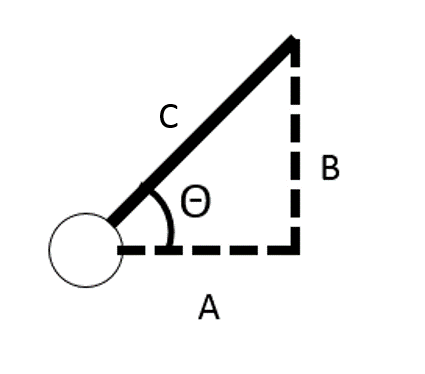# Physics problem

I want to try and set a bool to be true if a player object is higher than an angle on the x,z axis. Here is an image, so when the rigidbody falls, this bool sets to true.

Not sure which axis is which in your diagram, but what you’re looking for is called the inverse or arc tangent in trigonometry. In the image below, you can calculate the angle Theta in radians as `Math.Atan2(B, A);`. Remember that’s radians, so if you want to test if this is greater than 45 degrees let’s say, you would have `if(Math.Atan2(B, A) <= 45 * Math.PI / 180) { /* fallen code */ } `EDIT: I see now, you mean the angle against the XZ-plane. That’s a lot easier if you use the Sine rather than the tangent. the sine of an angle = opposite / hypotenuse, or `B / C` in our diagram (updated). So our formula is now

`if(Math.Asin(B / C) <= 45 * Math.PI / 180) { /* fallen code */ } `

If we use transform.forward as direction the object is facing, we can get the magnitude to use as C. B is then the Y component of that vector. So we have:

`if(Math.Asin(transform.forward.y / transform.forward.magnitude) <= 45 * Math.PI / 180) { /* fallen code */ } `

But transform.forward is already a normalized vector, which means its magitude is always 1. We can get rid of dividing by 1 to make the more simple equation:

`if(Math.Asin(transform.forward.y) <= 45 * Math.PI / 180) { /* fallen code */ } `